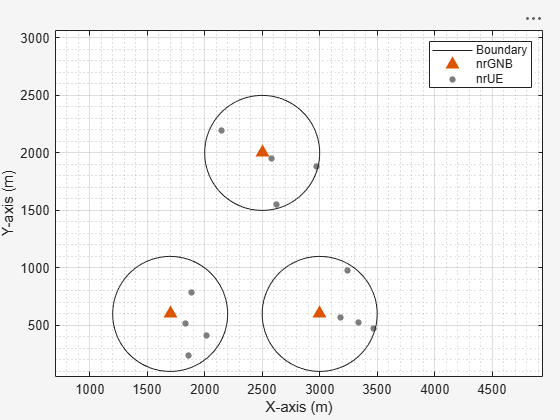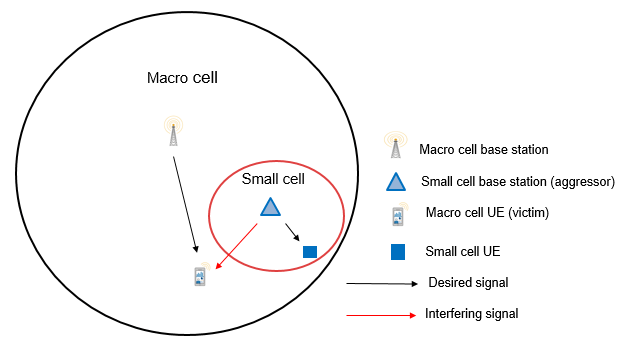# NR Intercell Interference Modeling

This example shows the impact on network performance due to downlink (DL) intercell interference caused by nearby cells. The example models a 5G New Radio (NR) network of multiple cells operating in the same frequency band. Each cell has a gNB placed at the center of the cell that serves a set of user equipment (UE). The NR stack on the nodes includes radio link control (RLC), medium access control (MAC), and physical layers (PHY).

### Introduction

This example models:

• Co-channel intercell interference.

• Slot-based round robin scheduling of physical downlink shared channel (PDSCH) resources.

• Free space path loss (FSPL) model.

• Single input single output (SISO) antenna configuration.

In this example, you consider the control packets, such as DL assignments, PDSCH feedback, and channel quality indicator (CQI) report, transmitted as out-of-band. Out-of-band refers to the transmission without the need for resources. It also refers to assured error-free reception.

### Co-Channel Interference

Co-channel cells are the NR cells operating on the same frequency. They can interfere with each other.

Consider this sample network topology consisting of 3 cells. Cell-1 and Cell-3 operate on the same frequency band. Cell-2 operates on a different frequency band and does not interfere with Cell-1 or Cell-3.### Scenario setup

Check if the Communications Toolbox Wireless Network Simulation Library support package is installed. If the support package is not installed, MATLAB® returns an error with a link to download and install the support package.

`wirelessnetworkSupportPackageCheck`

Create a wireless network simulator.

```rng("default") % Reset the random number generator numFrameSimulation = 20; % Simulation time in terms of number of 10 ms frames networkSimulator = wirelessNetworkSimulator.init;```

Specify the positions of 3 gNBs and the gNB of interest for visualizing metrics.

```gNBPositions = [1700 600 0; 3000 600 0; 2500 2000 0]; gNBOfInterestIdx = 3; % Specify a value between 1 and number of gNBs```

Create the gNBs. Specify the position, carrier frequency, channel bandwidth, subcarrier spacing, transmit power, and receive gain of each gNB. Each gNB operates one NR cell.

```gNBs = nrGNB(Position=gNBPositions,CarrierFrequency=2.5e9, ... ChannelBandwidth=10e6,SubcarrierSpacing=30e3,TransmitPower=32,ReceiveGain=11);```

Set the scheduler parameter `ResourceAllocationType` by using the `configureScheduler` function.

```for gNBIdx = 1:length(gNBs) % Resource allocation type value 0 indicate noncontiguous allocation of % frequency-domain resources in terms of RBGs configureScheduler(gNBs(gNBIdx),ResourceAllocationType=0) end```

Generate the positions of UEs in each cell.

```numCells = length(gNBs); cellRadius = 500; % Radius of each cell (in meters) numUEsPerCell = 4; uePositions = generateUEPositions(cellRadius,gNBPositions,numUEsPerCell);```

Create the UEs and connect them to a gNB. Configure full buffer traffic in the DL direction.

```UEs = cell(numCells,1); for cellIdx = 1:numCells ueNames = "UE-" + (1:size(uePositions{cellIdx},1)); UEs{cellIdx} = nrUE(Name=ueNames,Position=uePositions{cellIdx},ReceiveGain=11); connectUE(gNBs(cellIdx),UEs{cellIdx},FullBufferTraffic="DL") end```

Add gNBs and UEs to the network simulator.

```addNodes(networkSimulator,gNBs); for cellIdx = 1:numCells addNodes(networkSimulator,UEs{cellIdx}) end```

Get the cell ID for the gNB of interest. All the visualizations and metrics are shown for this cell.

`cellOfInterest = gNBs(gNBOfInterestIdx).ID;`

Set the `enableTraces` as `true` to log the traces. If the `enableTraces` is set to `false`, then traces are not logged in the simulation. To speed up the simulation, set the `enableTraces` to `false`.

`enableTraces = true;`

Create objects to log MAC and PHY traces.

```linkDir = 0; % Indicates DL if enableTraces simSchedulingLogger = cell(numCells,1); simPhyLogger = cell(numCells,1); for cellIdx = 1:numCells % Create an object for MAC DL scheduling traces logging simSchedulingLogger{cellIdx} = helperNRSchedulingLogger(numFrameSimulation, ... gNBs(cellOfInterest),UEs{cellOfInterest},linkDir); % Create an object for PHY layer traces logging simPhyLogger{cellIdx} = helperNRPhyLogger(numFrameSimulation,gNBs(cellOfInterest), ... UEs{cellOfInterest}); end end```

Update the output metrics plots periodically, specifying `numMetricsSteps` updates within the simulation. `numMetricsSteps` must be less than or equal to number of slots in simulation.

`numMetricsSteps = numFrameSimulation;`

Create an object for MAC and PHY metrics visualization.

```metricsVisualizer = helperNRMetricsVisualizer(gNBs(cellOfInterest),UEs{cellOfInterest}, ... CellOfInterest=cellOfInterest,NumMetricsSteps=numMetricsSteps, ... PlotSchedulerMetrics=true,PlotPhyMetrics=true,LinkDirection=linkDir);```

Write the logs to MAT-files. You can use these logs for post-simulation analysis.

`simulationLogFile = "simulationLogs"; % For logging the simulation traces`

Display the network topology.

`plotNetwork(cellOfInterest,cellRadius,gNBs,UEs);`Run the simulation for the specified `numFrameSimulation` frames.

```% Calculate the simulation duration (in seconds) simulationTime = numFrameSimulation*1e-2; % Run the simulation run(networkSimulator,simulationTime);```
```Custom channel model is not added. Using free space path loss (fspl) model as the default channel model. ``````gNBStats = statistics(gNBs); ueStats = cell(numCells, 1); for cellIdx = 1:numCells ueStats{cellIdx} = statistics(UEs{cellIdx}); end```

At the end of the simulation, the achieved value for system performance indicators is compared to their theoretical peak values (considering zero overheads). Performance indicators displayed are achieved data rate (DL), achieved spectral efficiency (DL), and BLER observed for UEs (DL). The peak values are calculated as per 3GPP TR 37.910. In the specified cell of interest, UE-1, UE-3, and UE-4 are almost equidistant from their gNB. However, you can observe lower throughput for UE-3 and UE-4 as compared to UE-1. This is because UE-3 and UE-4 experience higher intercell interference.

`displayPerformanceIndicators(metricsVisualizer)`
```Peak DL Throughput: 59.72 Mbps. Achieved Cell DL Throughput: 9.11 Mbps Achieved DL Throughput for each UE: [2.98 6.06 0.03 0.03] Peak DL spectral efficiency: 5.97 bits/s/Hz. Achieved DL spectral efficiency for cell: 0.91 bits/s/Hz Block error rate for each UE in the downlink direction: [0.752 0.501 0.992 0.992] ```

### Simulation Visualization

The run-time visualization shown are:

• Display of Network Topology: The figure shows the configured cell topology. For each cell, it shows the position of the gNB and the connected UEs.

• Display of DL scheduling metrics plots: For details, see 'Downlink Scheduler Performance Metrics ' figure description in NR Cell Performance Evaluation with MIMO example.

• Display of DL Block Error Rates: The plot displayed in 'Block Error Rate (BLER) Visualization' shows the BLER (for each UE) observed in the downlink direction, as the simulation progresses.

### Simulation Logs

The simulation logs are saved in MAT-files for post-simulation analysis and visualization. The per time step logs, scheduling assignment logs, and Phy reception logs are captured for each cell in the simulation and saved in the MAT-file `simulationLogFile`. After the simulation, open the file to load `NCellID`, `DLTimeStepLogs`, `SchedulingAssignmentLogs`, and PhyReception`Logs` in the workspace.

NCellID: This stores the cell ID and represents cell to which the simulation logs belong.

DL time step logs: Stores the per slot logs of the simulation with each slot as one row in the simulation. For details of log format, see the 'Time step logs' section of the NR Cell Performance Evaluation with MIMO example.

Scheduling assignment logs: Information of all the scheduling assignments and related information is logged in this file. For details of log format, see the 'Scheduling assignment logs' section in the NR Cell Performance Evaluation with MIMO example.

Phy reception logs: This file logs the packet reception information observed in the simulation. For details of log format, see the 'Phy reception logs' section in the NR Cell Performance Evaluation with MIMO example.

```if enableTraces % Get the logs simulationLogs = cell(numCells, 1); for cellIdx = 1:numCells if gNBs(cellIdx).DuplexMode == "FDD" logInfo = struct("NCellID",[],"DLTimeStepLogs",[], ... "SchedulingAssignmentLogs",[],"PhyReceptionLogs",[]); logInfo.DLTimeStepLogs = getSchedulingLogs(simSchedulingLogger{cellIdx}); else % TDD logInfo = struct("NCellID",[],"TimeStepLogs",[], ... "SchedulingAssignmentLogs",[],"PhyReceptionLogs",[]); logInfo.TimeStepLogs = getSchedulingLogs(simSchedulingLogger{cellIdx}); end logInfo.NCellID = gNBs(cellIdx).ID; % Get the scheduling assignments log logInfo.SchedulingAssignmentLogs = getGrantLogs(simSchedulingLogger{cellIdx}); % Get the Phy reception logs logInfo.PhyReceptionLogs = getReceptionLogs(simPhyLogger{cellIdx}); simulationLogs{cellIdx} = logInfo; end % Save simulation logs in a MAT-file save(simulationLogFile,"simulationLogs") end```

### Further Exploration

You can use this example to further explore these options:

• Model the uplink interference between the nodes by specifying the uplink-related configuration parameters. For details, see the NR Cell Performance Evaluation with MIMO example.

• Model the Aggressor-Victim scenarios: The aggressor is the source of interference and the victim suffers due to the interference. Consider the DL scenario in the following figure. The macro cell UE is far away from the macro base station (BS) and near to the small cell. In DL direction, macro cell UE suffers from interference by the small cell BS. The small cell BS is called the aggressor and macro UE is called the victim.• Model multiple clusters, where each cluster consists of cells operating on different frequencies, and analyze the impact of interference on the cell edge users.

### Local functions

```function plotNetwork(cellOfInterest,cellRadius,gNBs,UEs) %plotNetwork Create the network figure figure(Name="Network Topology Visualization",units="normalized", ... outerposition=[0 0 1 1],Visible="on"); title("Network Topology Visualization"); hold on numCells = numel(gNBs); for cellIdx = 1:numCells gNBPosition = gNBs(cellIdx).Position; % Plot the circle th = 0:pi/60:2*pi; xunit = cellRadius * cos(th) + gNBPosition(1); yunit = cellRadius * sin(th) + gNBPosition(2); if cellOfInterest == cellIdx h1 = plot(xunit,yunit,Color="green"); % Cell of interest else h2 = plot(xunit,yunit,Color="red"); end xlabel("X-Position (meters)") ylabel("Y-Position (meters)") % Add tool tip data for gNBs s1 = scatter(gNBPosition(1),gNBPosition(2),"^", ... MarkerEdgeColor="magenta"); cellIdRow = dataTipTextRow("Cell - ",{num2str(cellIdx)}); s1.DataTipTemplate.DataTipRows(1) = cellIdRow; posRow = dataTipTextRow('Position[X, Y]: ',{['[' num2str(gNBPosition) ']']}); s1.DataTipTemplate.DataTipRows(2) = posRow; % Add tool tip data for UEs uesPerCell = UEs{cellIdx}; for ueIdx = 1:numel(uesPerCell) uePosition = uesPerCell(ueIdx).Position; s2 = scatter(uePosition(1),uePosition(2),".",MarkerEdgeColor="blue"); s2.DataTipTemplate.DataTipRows(1) = uesPerCell(ueIdx).Name; posRow = dataTipTextRow('Position[X, Y]: ',{['[' num2str(uePosition) ']']}); s2.DataTipTemplate.DataTipRows(2) = posRow; end end % Create the legend if numCells > 1 legend([h1 h2 s1 s2],"Cell of interest","Interfering cells","gNodeB", ... "UE","Location", "northeastoutside") else legend([h1 s1 s2],"Cell of interest","gNodeB","UE","Location","northeastoutside") end axis auto hold off daspect([1000,1000,1]); % Set data aspect ratio end function uePositions = generateUEPositions(cellRadius,gNBPositions,numUEsPerCell) %generateUEPositions Return the position of UEs in each cell numCells = size(gNBPositions,1); uePositions = cell(numCells,1); for cellIdx=1:numCells gnbXCo = gNBPositions(cellIdx,1); % gNB X-coordinate gnbYCo = gNBPositions(cellIdx,2); % gNB Y-coordinate gnbZCo = gNBPositions(cellIdx,3); % gNB Z-coordinate theta = rand(numUEsPerCell,1)*(2*pi); % Expression to calculate position of UEs with in the cell. By default, % it will place the UEs randomly with in the cell r = sqrt(rand(numUEsPerCell,1))*cellRadius; x = round(gnbXCo + r.*cos(theta)); y = round(gnbYCo + r.*sin(theta)); z = ones(numUEsPerCell,1) * gnbZCo; uePositions{cellIdx} = [x y z]; end end```

### Appendix

The example uses these helper classes:

 3GPP TS 38.104. “NR; Base Station (BS) radio transmission and reception.” 3rd Generation Partnership Project; Technical Specification Group Radio Access Network.

 3GPP TS 38.214. “NR; Physical layer procedures for data.” 3rd Generation Partnership Project; Technical Specification Group Radio Access Network.

 3GPP TS 38.321. “NR; Medium Access Control (MAC) protocol specification.” 3rd Generation Partnership Project; Technical Specification Group Radio Access Network.

 3GPP TS 38.322. “NR; Radio Link Control (RLC) protocol specification.” 3rd Generation Partnership Project; Technical Specification Group Radio Access Network.

 3GPP TS 38.323. “NR; Packet Data Convergence Protocol (PDCP) specification.” 3rd Generation Partnership Project; Technical Specification Group Radio Access Network.

 3GPP TS 38.331. “NR; Radio Resource Control (RRC) protocol specification.” 3rd Generation Partnership Project; Technical Specification Group Radio Access Network.

 3GPP TR 37.910. “Study on self evaluation towards IMT-2020 submission.” 3rd Generation Partnership Project; Technical Specification Group Radio Access Network.